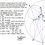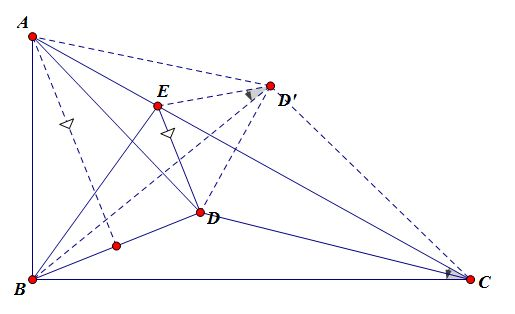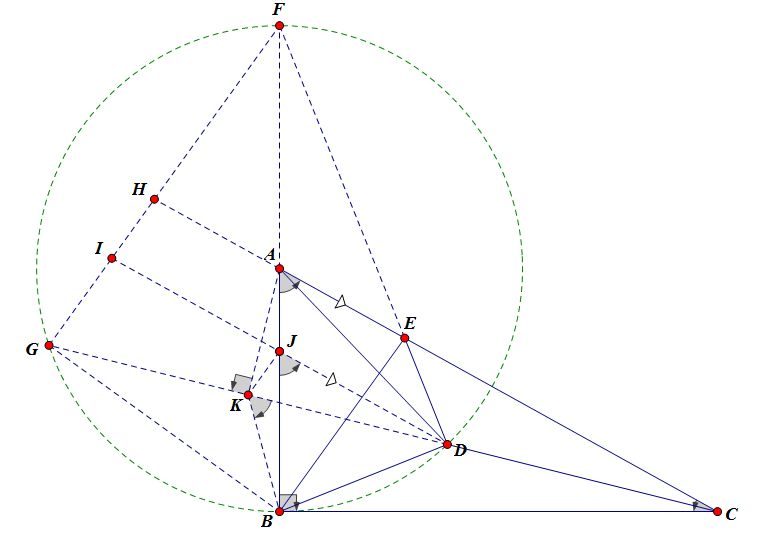# [Solutions Posted]Geometry Proof Problem of the day #1

I was browsing through my past photos and dug up an ample amount of geometry proof problems I've solved in the past. Starting today, I will select and share one of those problems and post it on here every few days. I hope you guys will find these problems interesting and fun.

This one caught my eye as the first problem:

$ABC$ is a right triangle at $B$. $D$ is a point inside $ABC$ such that $AD=AB$. $E$ is on $AC$ satisfying $DE\perp BD$. Suppose cirucmcircle $(CDE)$ intersects $BC$ at $F$. Prove that $BE=EF$.

Looking forward to solutions.involving different methods. Exercise your creativity on the limitless space of geo.Note by Xuming Liang
5 years, 9 months ago

This discussion board is a place to discuss our Daily Challenges and the math and science related to those challenges. Explanations are more than just a solution — they should explain the steps and thinking strategies that you used to obtain the solution. Comments should further the discussion of math and science.

When posting on Brilliant:

• Use the emojis to react to an explanation, whether you're congratulating a job well done , or just really confused .
• Ask specific questions about the challenge or the steps in somebody's explanation. Well-posed questions can add a lot to the discussion, but posting "I don't understand!" doesn't help anyone.
• Try to contribute something new to the discussion, whether it is an extension, generalization or other idea related to the challenge.
• Stay on topic — we're all here to learn more about math and science, not to hear about your favorite get-rich-quick scheme or current world events.

MarkdownAppears as
*italics* or _italics_ italics
**bold** or __bold__ bold
- bulleted- list
• bulleted
• list
1. numbered2. list
1. numbered
2. list
Note: you must add a full line of space before and after lists for them to show up correctly
paragraph 1paragraph 2

paragraph 1

paragraph 2

[example link](https://brilliant.org)example link
> This is a quote
This is a quote
    # I indented these lines
# 4 spaces, and now they show
# up as a code block.

print "hello world"
# I indented these lines
# 4 spaces, and now they show
# up as a code block.

print "hello world"
MathAppears as
Remember to wrap math in $$ ... $$ or $ ... $ to ensure proper formatting.
2 \times 3 $2 \times 3$
2^{34} $2^{34}$
a_{i-1} $a_{i-1}$
\frac{2}{3} $\frac{2}{3}$
\sqrt{2} $\sqrt{2}$
\sum_{i=1}^3 $\sum_{i=1}^3$
\sin \theta $\sin \theta$
\boxed{123} $\boxed{123}$

## Comments

Sort by:

Top Newest

Solution 1:We want to show that $\angle EBF = \angle EFB$. Since $\angle EFB = 180 ^ \circ - \angle EFC = 180^ \circ - \angle EDC$, it suffices to show that $\angle EBC + \angle EDC = 180 ^ \circ$.

We almost want to say that they are opposite angles of a cyclic quad, but $B, D$ lie on the same side of $EC$, and so that won't be possible. This motivates the construction of $D'$ as the reflection of $D$ in the line $EC$, and it suffices to show that $BED'C$ is a cyclic quad. There are many different approaches that we could use, and we will show that $\angle ECB = \angle ED'B$. We pick this angle because $\angle ACB$ is fixed in this question, while $D, E, D'$ are variable points.

Now, observe that $AB = AD = AD'$ so $A$ is the circumcenter of $\triangle B D D'$. This means that $\angle BD' D = \frac{1}{2} \angle BAD$. This motivates constructing $M$ as the midpoint of $BD$, so that we get $\angle BD'D = \angle BAM$. Hence, it suffices to show that $\angle ED'B + \angle BD'D = \angle ACB + \angle BAM$.

Observe that $AM \parallel ED$ since they are both perpendicular to $BD$. We then have $\angle ACB + \angle BAM = 90 ^ \circ - \angle MAC = 90^ \circ - DEC = \angle EDD'$. This is equal to $\angle ED'D$ due to isosceles triangle $ED'D$. Hence we are done.

- 5 years, 9 months ago

Log in to reply

Nice solution. +1

- 5 years, 9 months ago

Log in to reply

Solution 2:From the first paragraph of solution 1, we know it suffices to prove $180^{\circ}=\angle EDC+\angle EBC$.

Let $\omega$ denote the circle centered at $A$ with radius $AB$. Suppose $DE\cap \omega =F$ distinct from $D$, $CD\cap \omega =G$ distint from $D$. Since $ED\perp BD$, $FB$ is the diamenter and thus $F,A,B$ are collineaar.

The problem is equivalent to $\angle EBC=\angle FBG$ or $\angle GBE=90^{\circ}$. Since $FG\perp GB$, we only have to prove $BE\parallel GF$. Extend $AC$ to meet $GF$ at $H$, it now suffices to show $AE=AH$.(This is a well-known problem, proof is below)

Through $D$ construct a line parallel to $AC$ to intersect $FB,FG$ at $J,I$ respectively. By property of parallels $JD=IJ$ will imply $AE=AH$. To show this, let $K$ be the midpoint of $GD$, then we want to prove $JK||GI$.

Because $\angle FGD=\angle FBD$, the problem is now equivalent to $\angle JKD=\angle IGD=\angle FBD$ or $J,D,B,K$ are concyclic. Observe that $\angle AKC=90^{\circ}=\angle ABC$ since $K$ is the midpoint of chord $GD$, hence $A,K,B,C$ are concyclic. This means $\angle CKB=\angle CAB=\angle DJB$ by parallels and is sufficient to establish $JDBK$ is cyclic.

- 5 years, 9 months ago

Log in to reply

Solution 3(Simplest one yet): We want to prove $180-\angle EDC=\angle EBC$. The two angles seem too far away to be related, we attempt to bring them closer by constructing $X$ on $AC$ such that $BX\perp AC$. Since $AD^2=AB^2=AX*AC$, we know $ADC\sim AXD\implies \angle ADC=AXD$. Clearly $\angle ADE=90-\angle ADB=90-\angle ABD=\angle DBC$, Therefore the problem is equivalent to $180-\angle EDC-\angle ADE=\angle EBC-\angle DBC$ or $\angle CXD=\angle \angle EBD$, which is true because $BDEX$ is cyclic.

- 5 years, 9 months ago

Log in to reply

×

Problem Loading...

Note Loading...

Set Loading...